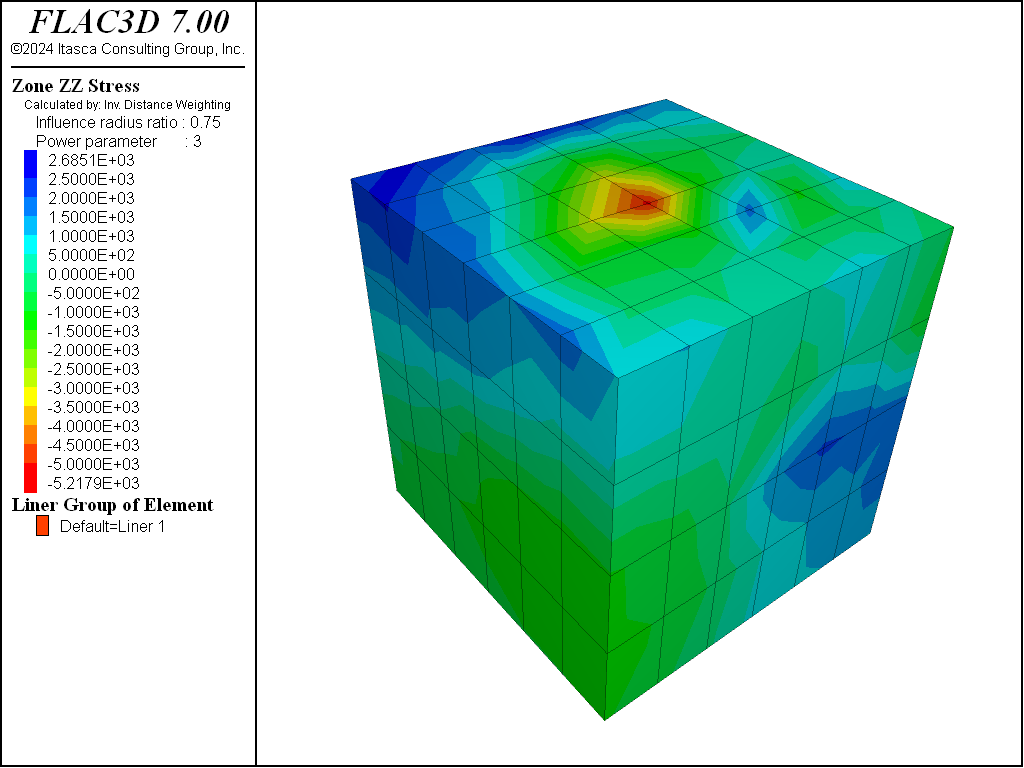# Demonstration of Large-Strain Sliding

Problem Statement

Note

The project file for this example is available to be viewed/run in FLAC3D. The main data files used are shown at the end of this example. The remaining data files can be found in the project.

A 0.33 m2 liner is placed on top of a one cubic meter soil block, moved down into the soil, and then moved laterally across the soil surface. The problem is run in large-strain mode. The liner is moved downward by 10 cm, and then it is moved laterally along the diagonal until the liner lies at the original corner of the top surface. For such large relative motion between the liner and the zones, it is necessary to set the large-strain sliding flag of the liner to on. (Liners interact with the grid via node-to-zone links. These links store the zone and an interpolation location within the zone to allow transfer of forces and velocities between the nodes and the zones. By default, these interpolation locations will not change, even if running in large-strain mode. The interpolation locations can be allowed to migrate through the grid by setting the large-strain sliding flag to structure liner property slide on.)

The FLAC3D model is a one cubic meter block of soil composed of 144 zones, with a 0.33 m2 concrete liner composed of 8 liner elements centered on top of the soil block, as shown in Figure 1. The soil is assigned elastic properties ($$E$$ = 15 MPa, $$\nu$$ = 0.3). The liner material is assigned properties corresponding with a concrete liner 0.1 m thick ($$E$$ = 25 GPa, $$\nu$$ = 0.15, $$t$$ = 0.1 m), and the following liner-zone interface properties are assigned:

 interface normal stiffness ($$k_n$$) 8 × 108 N/m3 interface shear stiffness ($$k_s$$) 8 × 108 N/m3 tensile strength ($$f_t$$) 0 MPa cohesion ($$c$$) 0 MPa residual cohesion ($$c_r$$) 0 MPa friction angle ($$\phi$$) 0 degreesFigure 1: FLAC3D model for demonstration of large-strain sliding.

First, the problem is run with large-strain sliding off by removing the structure liner property slide command from the data file. The $$zz$$-stresses in the zones after applying the first 10 cm downward displacement are shown in Figure 2. At this stage, the response is reasonable because large relative motion between the liner nodes and the zones has not occurred. However, after moving the liner along the diagonal, we see in Figure 3 that the $$zz$$-stresses and the zone deformation field no longer correspond with the liner location. This is incorrect. The interpolation locations have not moved from their original locations; therefore, the grid is still feeling a compressive force acting in the block center.

When the problem is rerun with large-strain sliding on, the behavior is correct (see Figure 4 and Figure 5). The $$zz$$-stresses and zone deformation field correspond with the liner location, because the interpolation locations have migrated through the grid to follow the liner motion. In fact, it is even possible to move the liner completely off the grid, as shown in Figure 6. If any liner nodes later come back into contact with any zone faces, then the corresponding links will be reestablished (see Figure 7).Figure 2: Vertical stresses in the soil (large-strain sliding off; normal displacement of 10 cm).Figure 3: Vertical stresses in the soil (large-strain sliding off; shear displacement of 47.6 cm).Figure 4: Vertical stresses in the soil (large-strain sliding on; normal displacement of 10 cm).Figure 5: Vertical stresses in the soil (large-strain sliding on; shear displacement of 47.6 cm).Figure 6: Vertical stresses in the soil (large-strain sliding on; shear displacement of 105 cm).Figure 7: Vertical stresses in the soil (large-strain sliding on; shear displacement of -453 cm).

Data File

LargeStrainSliding.dat

; SEL Liner example application
; Demonstration of Large-Strain Sliding for a Liner
model new
fish automatic-create off
model title 'Demonstration of Large-Strain Sliding for a Liner'

; Create the soil.
zone create brick size (6,6,4) point 0 (0,0,0) point 1 (1,0,0) ...
point 2 (0,1,0) point 3 (0,0,1)
zone cmodel assign elastic
zone prop bulk=12.5e6 shear=5.77e6  ; soil
zone face apply velocity-normal 0 range position-z 0

; Create the concrete liner.
struct liner create by-zone-face range position (0.33,0.33,1) (0.66,0.66,1)
struct liner property isotropic=(25e9,0.15) thickness=0.1  ; concrete
struct liner property coupling-stiffness-normal=8e8 ...
coupling-stiffness-shear=8e8 coupling-yield-normal=0.0 ...
coupling-cohesion-shear=0.0 ...
coupling-cohesion-shear-residual=0.0 coupling-friction-shear=0.0
struct node fix velocity  ; fully fix all nodes

struct mechanical damping combined-local
model large-strain on
zone history name='szz' stress-zz position (0.5,0.5,1)
model save 'slide0'

; Apply liner displacements - liner large strain-sliding off
struct node initialize velocity (0,0,-1e-4) local ; norm disp= -10 cm
model cycle 1000                                  ; shr  disp=   0
model save 'slide1-off'

struct node initialize velocity (3.3e-4,-3.3e-4,0.0) local ; norm disp=  0
model cycle 1000                                           ; shr  disp= +476 cm
model save 'slide2-off'

model restore 'slide0'
; Activate liner large strain-sliding
struct liner property slide on

; Apply liner displacements
struct node initialize velocity (0,0,-1e-4) local ; norm disp= -10 cm
model cycle 1000                                  ; shr  disp=   0
model save 'slide1'

struct node initialize velocity (3.3e-4,-3.3e-4,0.0) local ; norm disp=  0
model cycle 1000                                           ; shr  disp= +476 cm
model save 'slide2'
model cycle 1200                             ; shr  disp= +571 cm
model save 'slide3'

struct node initialize velocity (0,0,1e-3) local ; norm disp= +12 cm
model cycle 120                                  ; shr  disp=   0
struct node initialize velocity (-3.3e-3,3.3e-3,0.0) ; norm disp=  0
model cycle 315                                      ; shr  disp= -1500 cm
struct node initialize velocity (0,0,-1e-4) local ; norm disp= -12 cm
model cycle 1200                                  ; shr  disp=   0
model save 'slide4'


Endnotes

  To view this project in FLAC3D, use the program menu. Help ▼ Examples…   ⮡   FLAC     ⮡   Structure       ⮡   Liner         ⮡   LargeStrainSliding           ⮡   LargeStrainSliding.prj